## Chapter 4Fracture Mechanics and Delamination in Open TSVs

In Chapter 3 the nanoindentation technique was applied in order to locate the areas with a high concentration of mechanical stress in an open TSV. In these areas the probability of device failure, such as cracking or delamination, is high. The bottom of the TSV under consideration, which saw the highest stress build-up, consists of a multilayer structure which represents the Al-based interconnection used in CMOS technology. In this section we investigate how external forces, layer thicknesses, and the residual stress in the layers can induce delamination at the interfaces which form the bottom of an open TSV.

### 4.1 Basis of Fracture Mechanics

In order to understand the delamination failure it is first necessary to explain the theory of cracks in bodies. The following theories are based on and described in detail in . In continuum mechanics a crack is defined as a cut in a body which has its end inside the body volume. This end of the crack is referred to as a crack-tip. Crack surfaces are defined as the two opposite boundaries formed by the cutting plane and are generally treated as traction-free. The crack-tip and crack surfaces are illustrated in Figure 4.1.

In a solid material a crack can propagate in three different modes:

• Mode I: Opening the crack opens normal to the crack plane due to a load perpendicular to the crack surface.
• Mode II: Sliding the crack faces are displaced parallel to the crack surface and perpendicular to the crack-tip due to a shear loading.
• Mode III: Tearing the crack faces are displaced parallel to the crack surface and parallel to the crack-tip due to a shear loading acting in parallel to the crack tip.

These propagation modes can occur independently or in combination.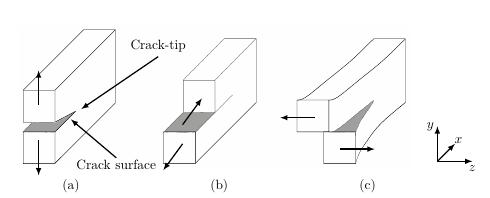Figure 4.1: The three crack opening modes. (a) indicates mode I (opening), (b) mode II (sliding) and (c) mode III (tearing).

To fully understand the crack propagation modes it is necessary to analyze the crack-tip field within a small region of radius R around the crack tip. Therefore the description of the crack-tip field demands the specification of a hybrid Cartesian and polar coordinate system as shown in Figure 4.2.

Figure 4.2: Coordinate at the crack-tip.

#### 4.1.1 Crack-Tip Field

The mechanical analysis of the crack-tip field under mode I (cf. Figure. 4.1 (a)) will be explained in the following and it is based on .

The derivation of the crack-tip field is achieved by employing the Airy stress function and the Westergaard approach which are described in Appendix A and Appendix B, respectively. The system analyzed is illustrated in Figure 4.2, where a set of in-plane Cartesian coordinates x and y, and polar coordinates r or φ at the crack tip are chosen. A complex representation z = x + iy of the coordinates is employed in the derivations.

A line crack with the following boundary conditions is considered:

1. Very large stresses at the crack tip.
2. Traction free crack surfaces.

The stresses σxx and σyy along the y = 0 plane can be expressed as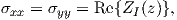(4.1)

where ZI(z) is the Westergaard function described in Appendix B and is employed to analyze crack problems . The Westergaard function permits to analyze the stress field at the crack tip by using a complex potential, as the Airy stress function, related to boundary condition of the system. In the following derivations the argument of Z is omitted for better readability and the subscript of Z indicates the crack mode. Because the strain energy in the body has to be finite, the order of the singularity of stresses at the crack has to be of higher order than z-12. Therefore the solution of the crack problem can be written in the form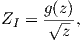(4.2)

where g(z) is a function which does not diverge at the crack tip (z 0). In this way the solution permits to obtain σyy →∞ for z 0, satisfying the first boundary condition. The second boundary condition σyy = 0 for x < 0 and y = 0, is defined by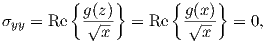(4.3)

which is satisfied if g(x) is real along y = 0.

The function g(z) close to the origin of the crack-tip can be represented by a Taylor series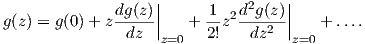(4.4)

Close to the crack-tip the function g(z) is real and constant. This constant g(0) is related to the stress intensity factor KI by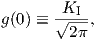(4.5)

which gives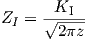(4.6)

close to the crack tip. The subscript of the stress intensity factor KI indicates the crack mode. K represents the magnitude of the crack tip stress.

The functions ZI and ZInecessary to describe the stresses near the tip can be expressed by employing the polar coordinate representation of z = re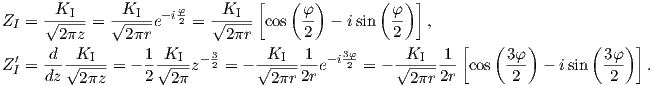(4.7)

Therefore the real and imaginary parts of the Westergaard function are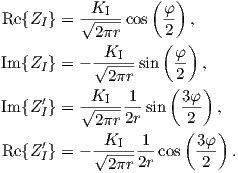(4.8)

The stresses at the crack-tip can be described by using the Westergaard function (more detail in Appendix B)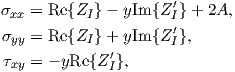(4.9)

where A is an uniaxial stress that does not add singularity at the crack tip. If we do not consider the higher-order terms, the stresses at the crack tip are given by plugging (4.8) into (4.9) (A is set to 0)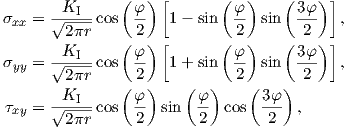(4.10)

where y = r sin φ = 2r sincos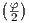.

The displacements at the crack-tip are obtained by following the same procedure (cf. Appendix B)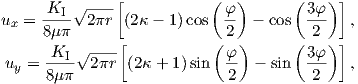(4.11)

where for the plane strain, κ and σzz are defined by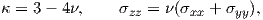(4.12)

and for the plane stress, they are defined by(4.13)

where μ denotes the shear modulus.

With (4.10) and (4.11) stresses and displacements at the crack-tip for mode I have been derived. These equations are valid for any cracked body under mode I, where if the stress σyy is know, the stress intensity factor for mode I can be obtained as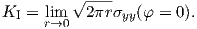(4.14)

Since the approach is analogous for the other two modes only the end results are given in the following. During mode II the stresses near the crack-tip are defined by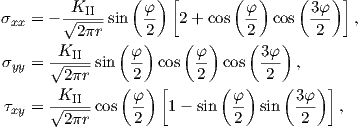(4.15)

and the displacements by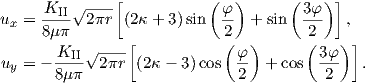(4.16)

If the stress τxy is known the stress intensity factor for mode II can be obtained as: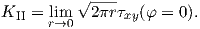(4.17)

For mode III the stresses near the crack-tip are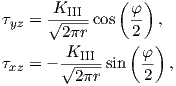(4.18)

and the corresponding displacement is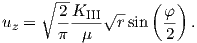(4.19)

Also here a stress intensity factor can be derived if the stress τyz is known(4.20)

#### 4.1.2 Delamination

In this section the fundamental equations required to describe cracks in the interface between two materials with different material properties, also referred to as delamination, are given. The reported theory is based on .

The concept of the intensity factor K, described in the previously section, cannot be easily applied, because the crack-tip in the case of delamination field has a different form compared to a crack in a single homogeneous material.

Figure 4.3: Tip of a bimaterial crack.

Delamination is illustrated in Figure 4.3. A bimaterial crack lies at the interface between two materials with elastic constants E1, ν1 and E2, ν2. This particular problem is analyzed by using an eigenfunction expansion method . For the description of the crack-tip field, a plane strain condition is considered, the complex variable method is employed (cf. Appendix A), and polar coordinates at the crack-tip are defined. The first boundary condition of the problem is that the crack surfaces (φ = ±π) are traction-free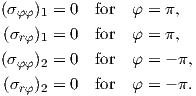(4.21)

The second boundary condition is the continuity of the stress field and the displacement field at the interface (φ = 0)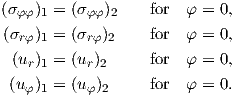(4.22)

These boundary conditions represent a system of equations where a general solution has to be obtained. One possible approach is to describe the problem through the Airy stress function ϕ for Material 1 and for Material 2. The Airy stress function satisfies the equilibrium equations (2.43) and therefore the compatibility equation (2.45) is given as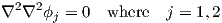(4.23)

with the Nabla operator 2 defined as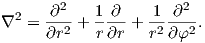(4.24)

To obtain the solution of the boundary conditions the variables of the stress function have to be separated in the following way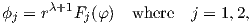(4.25)

where λ is the eigenvalue to be determined and Fj(φ) are the eigenfunctions of the biharmonic operator in (4.23.

If we insert (4.25) into (4.23), we obtain(4.26)

where the solution of the differential equations (4.23) can be obtained by using an Ansatz leading to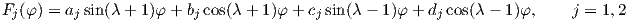(4.27)

where aj,bj,cj,dj are unknown constants.

By using (4.25) and the Airy stress in polar coordinates , the stress components are described by(4.28)

and the displacements in polar coordinates  by(4.29)

Equations (4.28) and (4.29) are further plugged into the boundary conditions (4.21) and (4.22)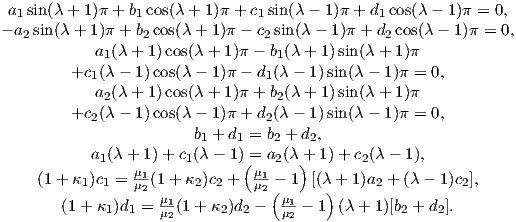(4.30)

A non-trivial solution of the system of equations (4.30) for the unknown coefficients aj,bj,cj, and dj exists if the determinant of the system 8×8 vanishes. The solution of the system of equations leads to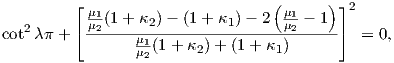(4.31)

where this equation only has complex solutions for λ (real solution results only if a homogeneous material is considered Material 1 = Material 2). Therefore a physically solution  is obtained for the set of eigenvalues: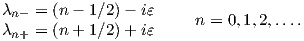(4.32)

where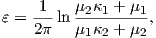(4.33)

with μi = Ei2(1 + νi), κi = 3 - 4νi and ε the bimaterial constant.

The field which dominates as r 0 (forming a singularity of the stress at the crack-tip) corresponds to the eigenvalue with the smallest real part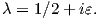(4.34)

Taking into account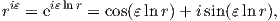(4.35)

and using (4.28) and (4.29), and considering (4.34) the stresses and displacements can be approximated to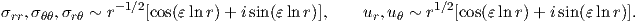(4.36)

It is clear that the typical 1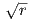-type singular behavior for the stresses and the displacements is present at the bimaterial crack-tip. Unlike for a homogeneous body, these quantities oscillate, with increasing amplitude, while approaching the crack-tip.

The crack tip stresses (in Cartesian coordinates) can be expressed by introducing the stress intensity factors  as: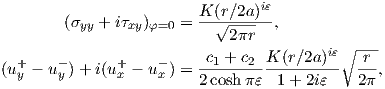(4.37)

where 2a indicates an arbitrary reference crack length, the sign + and - are the upper and lower surface of the crack tip, respectively (Figure 4.3), and K is the stress intensity factor which can be separated in a real and an imaginary part: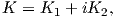(4.38)

and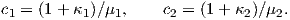(4.39)

The stress intensity factors and the length 2a are weighted by the material constant ε and therefore a decomposition into purely mode I and mode II is not possible. This can be seen if using relation (4.35) we write the stresses at the interface from (4.37) in the following form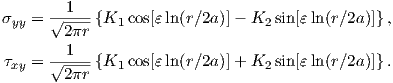(4.40)

The stress intensity factor K1 is associated with the normal and the shear stresses in the interface, which is also true for K2 since during delamination both modes are inseparably connected to each other. In the homogeneous material case K1 and K2 correspond to K

IandK_II,respectively.

#### 4.2.0 Energy Release Rate

The energy release rate G is a fundamental concept in fracture mechanics . This quantity is the released energy dΠ during an infinitesimally small crack advance dA (A indicates a crack area in 3D and a crack length in 2D)(4.41)

The total potential energy Π is composed of the strain energy U and the potential of external forces V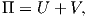(4.42)

where U is defined by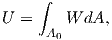(4.43)

where A0 is the initial area of the crack. V is defined by(4.44)

W in (4.43) is the strain energy density given by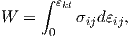(4.45)

where σij denotes the components of the stress tensor and εij the components of the strain tensor . In (4.44) Ti indicates a prescribed traction on Γt and ui the corresponding displacements (cf. Figure 4.4). If the body is not subjected to external tractions the potential energy is equal to the strain energy Π = U.

Figure 4.4: Example of crack advance in two-dimensional.

In two-dimensional problems, dΠ is related to the crack extension and therefore by considering an infinitesimally small crack extension da, G is defined by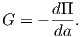(4.46)

For a homogeneous material and assuming the linear elastic case G can be expressed in terms of stress intensity factors, where G can describe a single mode or a combination of modes.

We derive G for mode I (cf. Figure 4.1 (a)), where the stress before the crack elongates is given by σyy and the displacement after the crack has elongated is given by uy along y = 0. The strain energy generated over an increment of crack extension of length Δa is given by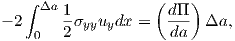(4.47)

where the minus sign indicates that the stresses and displacements act in opposite directions during relaxation and the factor of 2 refers to the two crack surfaces. By using the definition of G (cf. (4.46)) and by considering a crack extension of a small length Δa in the limit Δa 0, the energy release rate for mode I is calculated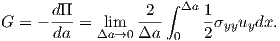(4.48)

In the current problem, (4.6) is employed to describe the stress and displacement of interest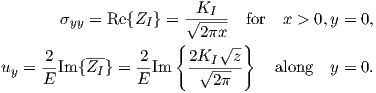(4.49)

It is necessary to consider the displacement of interest which corresponds to the new crack length. Therefore x = Δa and z = -(x- Δa) + iy,y = 0; therefore, the displacement of interest is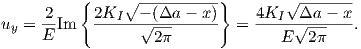(4.50)

By plugging the stress (4.49) and the displacement (4.50) into (4.48) G can be obtained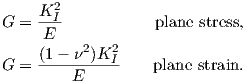(4.51)

For modes II and III the same derivation can be carried out, leading to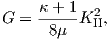(4.52)

for mode II and to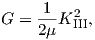(4.53)

for mode III. If a general crack loading is taken in consideration, all three modes are present and G is described by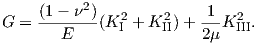(4.54)

For delamination, G can derived by applying the same approach resulting in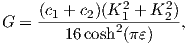(4.55)

where c1 and c2 are described by (4.39), and ε by (4.33). Here G is uniquely determined by both stress intensity factors K1 and K2.

#### 4.2.1 Failure Criterion

During the fracture process new surfaces are generated . During the crack process the material is separated along the fracture surface dA, which can be divided into the upper dA+ and lower dA- surface of the crack. During the creation of a crack expansion of dA, the energy required to generate the fracture is given by dΓ. During the material separation due to cracking dΓ is given by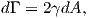(4.56)

where γ is the fracture surface energy usually considered to be constant and the factor of 2 stands for the two surfaces of the crack.

If we consider a quasi static fracture process of an elastic body the balance of the energy in play reads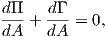(4.57)

thus during the fracture process the change of the potential Π due to an external or internal force and the fracture energy Γ sum up to zero. Using (4.56) and (4.41) we can write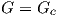(4.58)

with Gc = 2γ. Expression (4.58) can be understood as a condition for crack propagation. If the released energy is equal to the energy needed for the fracture process the crack will advance. This fracture criterion is know as Griffith’s fracture criterion  and it is applicable for homogeneous materials. For the relation between G and Ki (i indicates the crack mode) this criterion can be used for any combination of crack modes.

The fracture criterion can also be described for bimaterial cracks. In Section 4.1.2 it was shown that during delamination mode I and mode II always act together. G depends on K1 and K2 and therefore the fracture criterion is based on both modes. The phase angle Ψ of the mixed mode characterizes G; therefore, the fracture criterion is described as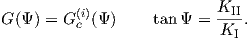(4.59)

The term Gc(i)(Ψ) is the fracture toughness and is a function of the phase angle Ψ. The fracture resistance of the interface has to be experimentally measured.

### 4.3 Energy Release Rate Calculation

The evaluation of the energy release rate G can be used to predict the crack or delamination propagation. In this section the various procedures to calculate G are described. These methodologies are then applied to the TSV structure in Section 4.4 and Section 4.5.

#### 4.3.1 J-Integral

In linear fracture mechanics the value G can be obtained by applying the J-Integral method .

The value of the J-Integral is equal to the energy which is dissipated by the fracture. The J-Integral method is applicable for systems obeying linear-elastic fracture mechanics as well as for materials with an inelastic behavior. The J-Integral is evaluated along a path Γ around the crack-tip of the cracked body. The path can be arbitrary chosen as long as the crack-tip is inside the region surrounded by the path .

The definition of the J-Integral is given by(4.60)

where W is the strain energy density, Ti are the components of the traction vector, ui are the components of the displacement vector, and ni are the components of the unit vector perpendicular to the integration path (Figure 4.5). The strain energy density is the work per unit volume done during the elastic deformation of a material and is defined by (4.45).

Figure 4.5: A possible (arbitrary) path around the crack-tip for the J-integral.

For linear elastic materials, introducing (2.37) into (4.45), W is defined by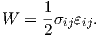(4.61)

The traction vector is defined by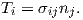(4.62)

Considering a straight bond line, the standard J-Integral, primarily developed for problems of single homogeneous materials, can also be applied to bi-material interfaces .

#### 4.3.2 Regression Analysis

The energy release rate G can be calculated by determining the values K1 and K2 from (4.55). These values are obtained by regression analysis fitting. Regression analysis permits to obtain unknown parameters by employing fitting functions. One of the most common approaches is the least squares method, where through the minimization of the sum of squared residuals (residual is the difference between an observed value and the fitted value provided by a model) the fitting parameters can be obtained .

The stress intensity factors are obtainable by using the stress at the head of the right side of the crack-tip (cf. Figure 4.3) which is described by (4.40). The equation for σyy is multiplied by cos[εln(r∕2a)] and added to the equation for τxy previously multiplied by sin[εln(r∕2a)] . The resulting equation is denoted as σ1 and represents the combined stress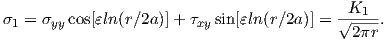(4.63)

An additional combined stress σ2 can be obtained by multiplying τxy with cos[εln(r∕2a)]. An addition to the product of σyy and sin[εln(r∕2a)] leads to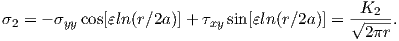(4.64)

The values σ1 and σ2 can be calculated using σyy and τxy, which can be obtained from FEM simulations. The stress intensity factors K1 and K2 are calculated by a regression of σ1 versus r and σ2 versus r . The values K1 and K2 can be further used to calculate G using (4.55).

### 4.4 Delamination Prediction at TSV Bottom

In this section the J-Integral method was employed to predict delamination failure at the TSV bottom. The study was carried out by means of simulation, which is based on the evaluation of the J-Integral at different interfaces. These simulations enable the determination of the structures with the lowest failure probability.

The study of delamination in TSVs is necessary, because the delamination can increase the probability of cracking or corrosion of the conducting layer (W) or lead to rupture of sidewall oxide isolation. To limit these problems different factors were analyzed to understand how they can influence delamination, and the mechanical stability of the device.

#### 4.4.1 Studied System

The existence of mechanical stress can be sufficient to degrade the performance and to induce crack or delamination in the TSVs . In Section 3 the critical areas where a mechanical failure of the TSV can be assumed were found. One of these areas is the bottom of the TSV sidewall which consists of various interfaces between different material films with different thicknesses and mechanical properties. At these interfaces the possibility of delamination leading to failure of the device needs to be considered.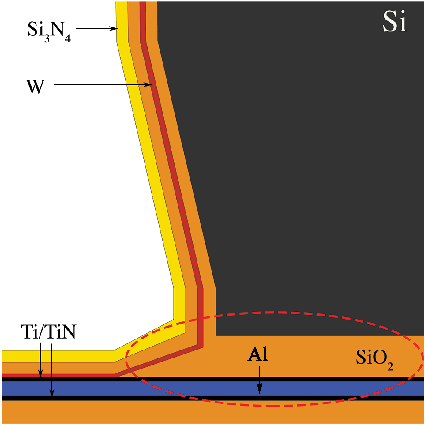Figure 4.6: Schematic of the Open TSV geometry under consideration. The open TSV is integrated in the silicon of the wafer. The alternating layers with different thickness are located at the bottom. There are the interfaces where a failure of the device due to delamination is expected.

Figure 4.6 depicts the open TSV studied . Different factors such as the residual stress of the films, the film thicknesses, and the external forces influence the failure of the device. By analyzing these factors the critical mechanical and geometrical conditions which influence G, and thereby the probability of delamination, can be studied.

#### 4.4.2 Approach

The energy release rate G was calculated for the different interfaces at the bottom of the TSV by using COMSOL Multiphysics . Considering the bottom of an open TSV as free to bend, cracking or delamination of the layers has to be expected under the sidewall (cf. red circled region Figure 4.6).

Figure 4.7: Schematic representation of the studied system. In the inset the path Γ for the J integral calculation is shown. The variable a indicates the crack length and w the width of the layer.

Two-dimensional simulations for the structure shown in Figure 4.7 were carried out. All the layers have a length w of 20 μm and the thicknesses of the layers were varied according to the values given in Table 4.1.

All the materials were assumed to be linear elastic and isotropic. The failure of the interconnection in the area under the sidewall was studied; therefore, the top layer of the simulation space was assumed mechanically fixed as the sidewall of the TSV was fixed. Furthermore, a downward force was applied on the bottom of the system and the bottom of the TSV was considered free to bend (cf. Figure 4.7). The simulations were started with a small initial crack length a and gradually increased until a predefined value was reached.

The four interface systems found in the open TSV are Ti/Al, SiO2/TiN, SiO2/W, and Si/SiO2. For the prediction of failure due to delamination, the values G are compared to critical values Gc taken from  and showed in the Table 4.2.

Calculations were carried out for different ratios of crack length a and layer width w. All simulations start with a crack of length 0.5 μm which was gradually increased by steps of 0.5 μm until 3 μm was reached.

 Layer Ti/TiN SiO2 Al W Si Thickness (μm) 0.05-0.2 0.3-1.4 0.3-0.6 0.04-0.16 5

Table 4.1: Thickness of layers employed.

 Interface SiO2/TiN Si/SiO2 SiO2/W Gc (J/m2) 1.9 1.8 0.2-0.5

Table 4.2: Critical values Gc for the considered interface.

By varying the following factors their influence on G was investigated:

• Residual stress: The stress was simulated by adding an initial stress in each considered layer. It was introduced by setting σxx and σyy to the assumed stress values. The residual stress in the layer is due to the combination of the film deposition process and thermal processes. Small changes in residual stress influence the value of G.
• Thicknesses of the layers: The thickness of the layers influence G and different values were employed to find the critical condition for the delamination.
• External force: It can represent extra mechanical stress during the fabrication processes (for example, during bonding between dies or between the interposer layer and die ), or an accidental load, for example due to the presence of dust particles.

G was calculated by employing the J-Integral method (Section 4.3.1) where the integral path Γ is displayed in Figure 4.7. Because the experimental critical values of Gc found in literature have a larger range of phase angles Ψ and because the main mode which acts in the considered structure is unknown, only G was evaluated in this study, without investigate the value of Ψ. Therefore, the failure prediction is made by comparing G from the simulations with Gc.

#### 4.4.3 Results

In all the plots shown (Figures 4.8-4.23), the x-axis represents the ratio a∕w, the y-axis the considered factors, and the z-axis the calculated G. For the residual stress and thickness analyses a downward force of 110 mN was applied (F in Figure 4.7).

##### 4.4.3.1 Residual Stress Analysis

In Figure 4.8 the G values for the interface between Ti and Al are plotted.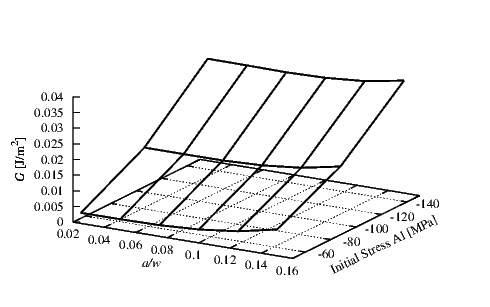Figure 4.8: The energy release rate G for different initial stresses (Al) and crack lengths in the interface Ti/Al. Simulation conditions were σTi=-50 MPa, hTi=0.15 μm, and hAl=0.5 μm.

The initial stress in the Al was assumed to be compressive. Inside the Ti a constant compressive initial stress σTi of 50 MPa was used. For the Ti a thickness hTi of 0.15 μm and for the Al a thickness hAl of 0.5 μm were set. The effect of the ratio a∕w on G is small compared to the influence of the initial stress. This shows that the probability of a failure is effectively reduced by a decrease in the initial stress in the Al layer.

Figure 4.9 depicts the G value for an interface between Ti and Al where the initial stress in the Ti was varied. For the Ti a thickness hTi of 0.15 μm and for the Al a thickness hAl of 0.5 μm were set. A compressive stress σAl of 100 MPa was set in the Al layer. A reduction in the initial stress in Ti leads to an increase in the energy release rate; therefore, in contrast to Figure 4.8 the decrease in the initial stress in the Ti layer can lead to a delamination propagation. The increase of the crack length does not significantly influence G. For this interface no value of Gc to compare with our values were available in literature. Nevertheless the G values are very small thus we can assume no delamination failure for this interface.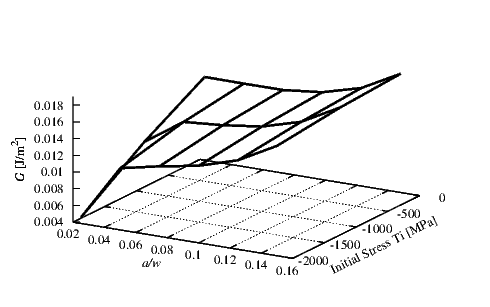Figure 4.9: The energy release rate G for different initial stresses (Ti) and crack lengths in the interface Ti/Al. Simulation conditions were σAl=-100 MPa, hTi=0.15 μm, and hAl=0.5 μm.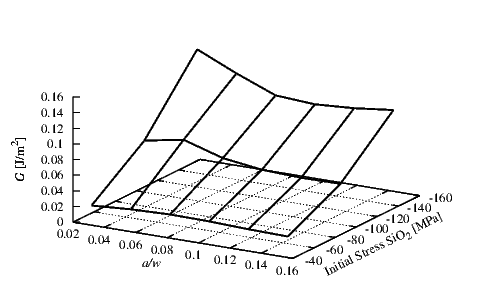Figure 4.10: The energy release rate G for different initial stresses (SiO2) and crack lengths in the interface Si/SiO2. Simulation conditions were hSi=5 μm, and hSiO 2=1.4 μm.

Figure 4.10 shows the behavior of G at the interface between Si and SiO2. Thicknesses of 5 μm and 1.4 μm for Si (hSi) and SiO2 (hSiO 2) were used, respectively. The critical value Gc was found to be 1.8 J/m2 . All the points are far below the critical value. A slight decrease of G with an increase of the crack length is observable. This shows the stability of this interface for every crack length and for every initial stress in the SiO2 layer.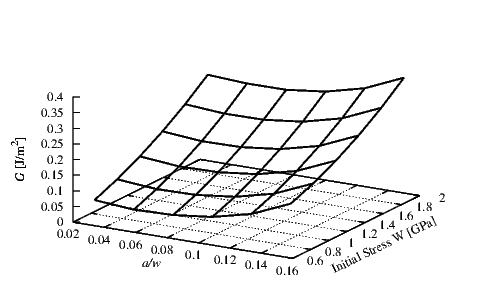Figure 4.11: The energy release rate G for different initial stresses (W) and crack lengths in the interface SiO2/W. Simulation conditions were σSiO 2=-100 MPa, hW=0.1 μm and hSiO 2=0.4 μm.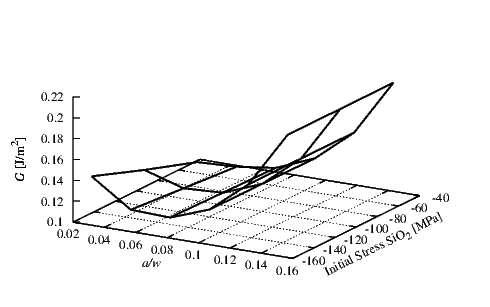Figure 4.12: The energy release rate G for different initial stresses (SiO2) and crack lengths in the interface SiO2/W. Simulation conditions were σW=1.25 GPa, hSiO 2=0.4 μm, and hW=0.1 μm.

The behavior of G at the SiO2/W interface is shown in Figure 4.11 for thicknesses of 0.4 μm and 0.1 μm for the SiO2 (hSiO 2) and the W (hW) layers, respectively. In the SiO2 an initial compressive stress σSiO 2 of 100 MPa was assumed. The simulations were carried out for different tensile initial stresses in the W layer. The Gc according to  is in the range of 0.2-0.5 J/m2 and therefore quite small compared to G obtained for the SiO2/W interface. For this system the influence of the a∕w ratio variation is small compared to the initial stress variation. There is a constant increase in G with an increase in the initial tensile stress in the W layer. Initial stresses above 1.25 GPa will lead to delamination at all a∕w ratios and therefore to the failure of the device.

In Figure 4.12 the interface SiO2/W was also taken in consideration. An initial tensile stress σW of 1.25 GPa and a thickness hW of 0.1 μm were used for the W layer. Different initial compressive stresses in the SiO2 using a thickness hSiO 2 of 0.4 μm were simulated. An increase in G is observable for increasing crack lengths a. In contrast to the behavior of G due to a varying initial stress in the W layer, here the variation of G with respect to the variation of the initial stress in the SiO2 is negligible. Only at high crack lengths is the modeled G value close to the critical Gc. Therefore, the main influence to the stability of this interface is connected to the crack length.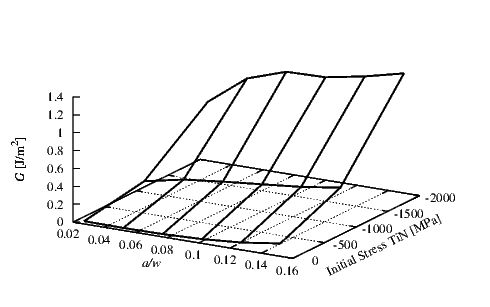Figure 4.13: The energy release rate G for different initial stresses (TiN) and crack lengths in the interface SiO2/TiN. Simulation conditions were σSiO 2=-100 MPa, hSiO 2=1 μm, and hTiN=0.15 μm.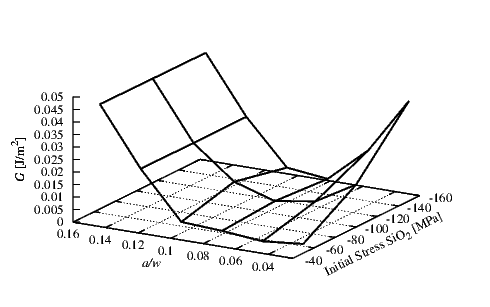Figure 4.14: The energy release rate G for different initial stresses (SiO2) and crack lengths in the interface SiO2/TiN. Simulation conditions were σTiN=-50 MPa, hSiO 2=1 μm, and hTiN=0.15 μm.

Figure 4.13 shows the behavior of G at the interface SiO2/TiN. Here thicknesses of 1 μm and 0.15 μm for the SiO2 (hSiO 2) and TiN (hTiN) were used, respectively. A compressive stress σSiO 2 of 100 MPa was used for the SiO2 layer. Critical value Gc for this interface was found to be 1.9 J/m2 . In this configuration the a∕w ratio does not cause failure at the interface. The main effect which can be the cause of problems in this interface is the initial stress in the TiN. A constant increase of G related to the initial stress can be observed.

In Figure 4.14 the SiO2/TiN interface was analyzed. The thickness of 1 μm for the SiO2 (hSiO 2) layer and 0.15 μm for the TiN (hTiN) layer with a compressive initial stress σTiN of 50 MPa for the TiN were chosen. By varying the a∕w ratio in the range 0.08 to 0.1 a minimum G at high initial stresses is found. This behavior results in a high G for short and long crack lengths. This means that the presence of a crack in the interface does not support the propagation, provided that the length of the initial crack does not exceed a certain critical value. Under the modeled variation configuration and load delamination will not occur as the values of G are below the critical value.

##### 4.4.3.2 Thickness Analysis

In Figures 4.15-4.19 the calculated G for different layers thicknesses is shown. In this section the influence of the layer thicknesses are investigated. The results for the SiO2/W interface are presented in Figure 4.15. For the SiO2 layer a thickness hSiO 2 of 0.4 μm and a compressive stress σSiO 2 of 100 MPa were used. In the W layer a tensile stress σW of 1.25 GPa was applied. The effect of the W thickness is highly relevant because a high increase of G is observed at long crack lengths and small thicknesses. For large thicknesses of W the calculated G is in the range of the Gc and for this condition we can expect delamination to take place.Figure 4.15: The energy release rate G for different thicknesses (W) and crack lengths in the SiO2/W interface. Simulation conditions were σSiO 2=-100 MPa, σW=1.25 GPa, and hSiO 2=0.4 μm.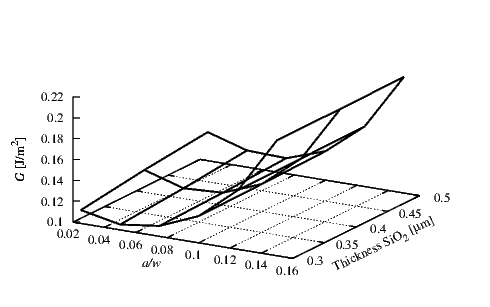Figure 4.16: The energy release rate G for different thicknesses (SiO2) and crack lengths in the SiO2/W interface. Simulation conditions were σSiO 2=-100 MPa, σW=1.25 GPa, and hW=0.1 μm.

Figure 4.16 shows the behavior of G at the SiO2/W interface. Here a thickness hW of 0.1 μm for the W was set. A compressive stress of 100 MPa in the SiO2 (σSiO 2) layer and a tensile stress of 1.25 GPa in the W (σW) layer were used. The thickness of the SiO2 does not appear to have a significant influence on G and the increase in G is only attributed to the a∕w ratio.

In Figure 4.17 the results at the interface between SiO2 and TiN for different TiN thicknesses are displayed. A layer thickness hSiO 2 of 1 μm and a compressive initial stress of 100 MPa for the SiO2 (σSiO 2) and a compressive initial stress of 50 MPa in the TiN (σTiN) were chosen. For this interface at very small thicknesses and at long crack lengths there is an important increase in G which can exceed the critical Gc of 1.9 J/m2 .

In Figure 4.18 the interface between the Ti and Al for different thicknesses were studied. A thickness hTi of 0.15 μm and a compressive initial stress σTi of 50 MPa for the Ti was used. A compressive stress σAl of 100 MPa for the Al was chosen. The critical energy release rate for this interface is not available in literature. We can assume that the delamination will not appear because the calculated values of G are very small. A significant increase in G is noted only at small thicknesses of the Al and at high crack lengths. For the other configurations the G remains almost unchanged.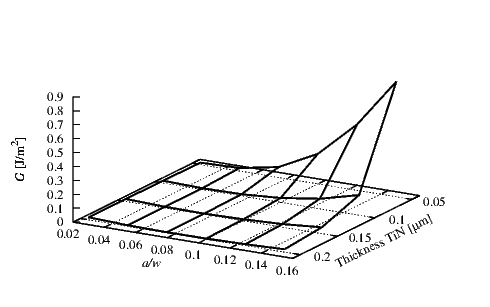Figure 4.17: The energy release rate G for different thicknesses (TiN) and crack lengths in the SiO2/TiN interface. Simulation conditions were σSiO 2=-100 MPa, σTiN=-50 MPa, and hSiO 2=1 μm.

In Figure 4.19 the G values of the interface between Si and SiO2 are reported. An initial compressive stress σSiO 2 of 100 MPa in the SiO2 was used. For the Si layer a thickness hSi of 5 μm was set. The thickness variation of the SiO2 does not produce high value of G.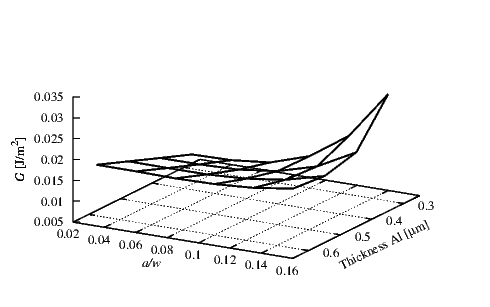Figure 4.18: The energy release rate G for different thicknesses (Al) and crack lengths in the Ti/Al interface. Simulation conditions were σTi=-50 MPa, σAl=-100 MPa, and hTi=0.15 μm.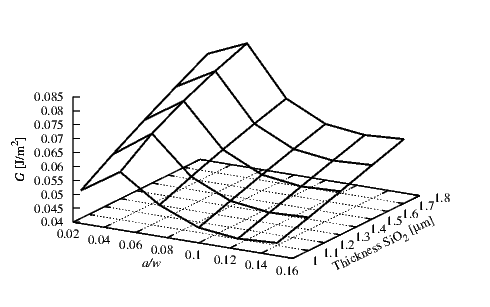Figure 4.19: The energy release rate G for different thicknesses (SiO2) and crack lengths in the Si/SiO2 interface. Simulation conditions were σSiO 2=-100 MPa and hSi=5 μm.

##### 4.4.3.3 Force Analysis

The effects of different forces on the system are presented in Figures 4.20-4.23. In these simulations different forces in the range of 10-210 mN were applied.

The behavior of G at the SiO2/W interface is shown in Figure 4.20. A thickness of 0.4 μm for the SiO2 (hSiO 2) layer and a thickness of 0.1 μm for the W (hW) layer were employed. In the SiO2 a compressive initial stress σSiO 2 of 100 MPa and in the W a tensile stress σW of 1.25 GPa were applied. The simulations were carried out for different forces. The Gc is in the range of 0.2-0.5 J/m2  and therefore small in comparison to the values of G obtained for the SiO2/W interface. For this system delamination is expected only when a force over 100 mN is applied.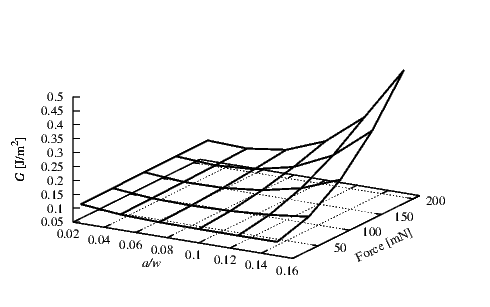Figure 4.20: The energy release rate G for different force and crack lengths in the SiO2/W interface. Simulation conditions were σSiO 2=-100 MPa, σW=1.25 GPa, hSiO 2=0.4 μm, and hW=0.1 μm.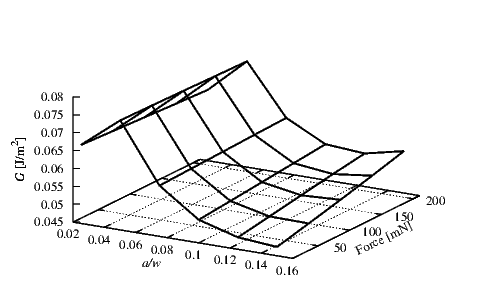Figure 4.21: The G for different forces and crack lengths in the Si/SiO2 interface. Simulation conditions were σSiO 2=-100 MPa, hSiO 2=1.4 μm, and hSi=5 μm.

In Figure 4.21 the G values of the interface between Si and SiO2 are plotted against the a∕w ratio. An initial compressive stress σSiO 2 of 100 MPa in the SiO2 with a thickness hSiO 2 of 1.4 μm were used. For Si a layer thickness hSi of 5 μm was set. The effect of a force variation is small compared to the influence of the a∕w ratio. In this interface for smaller crack lengths the values of G are larger than for long crack lengths. The critical Gc value of 1.8 J/m2  for this interface is much larger than those calculated in the given simulations.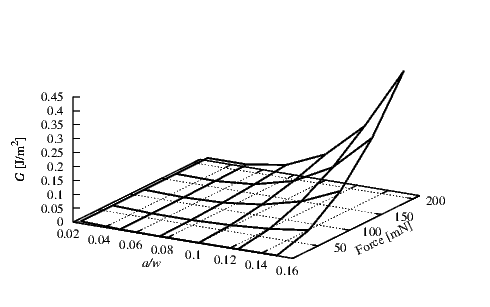Figure 4.22: The G for different forces and crack lengths in the SiO2/TiN interface. Simulation conditions were σSiO 2=-100 MPa, σTiN=-100 MPa, hSiO 2=1 μm, and hTi=0.15 μm.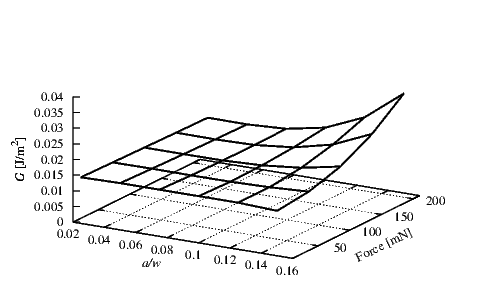Figure 4.23: The G for different forces and crack lengths in the Ti/Al interface. Simulation conditions were σTi=-50 MPa, σAl=-100 MPa, hTi=0.1 μm, and hAl=0.5 μm.

The G values of the interface between the SiO2/TiN are shown in Figure 4.22. Thicknesses of 1 μm and 0.15 μm for the SiO2 (hSiO 2) and TiN (hTi) were used, respectively. A compressive stress of 100 MPa for the SiO2 (σSiO 2) and 100 MPa for the TiN (σTiN) were applied. Here G increases with the increase in the crack length and the applied force. The calculated values of G are much lower than Gc, therefore we can expect delamination only for large applied forces.

In Figure 4.23 the G values obtained for the interface between the Ti/Al are reported. For the Ti layer a thickness hTi of 0.1 μm and initial compressive stress σTi of 50 MPa were employed. The Al layer was assumed to have a thickness hAl of 0.5 μm and an initial compressive stress σAl of 100 MPa. The G values obtained are very small therefore no delamination can be supposed for this interface.

#### 4.4.4 Discussion

The impact of the layer thicknesses, the residual stresses, and the applied forces on the energy release rate G were investigated and are summarized in Table 4.3.

In Section 4.4.3.1 the effects of the films’ residual stresses on G were investigated. In the SiO2/W interface, a high probability of delamination propagation occurs when, due to the deposition process and thermal processes, the W layer exhibits high values of residual stress. In addition, the presence of defects, generated during film deposition and resulting in the presence of cracks at the interface, increase the probability of delamination failure. The SiO2/TiN interface shows the possibility of delamination propagation only for high values of compressive initial stress in the TiN layer. The variation of initial stress in the SiO2 does not produce high G values decisive for mechanical failure. The Si/SiO2 interface remains stable for every crack length and residual stress. Although the critical energy release rates are not available in literature for the Ti/Al interface, a qualitative estimation of the delamination can be given. As the obtained energy release rate is very small, no delamination propagation in this interface is anticipated. In the SiO2/W interface the most critical condition that lead to delamination propagation is obtained when high values of residual stress in the W layer are noted.

In Section 4.4.3.2 the effects of the layer thicknesses were investigated. Different values of thicknesses of the layer change the value of G. From simulations it is possible to conclude that for long crack lengths the thickness of the layer has an important effect on the stability of the interface. Usually a thickness decrease strongly increases G. This is not applicable for the SiO2/W interface, where also at high thicknesses a high G was obtained.

In Section 4.4.3.3 the effects of an external load were studied. The increased applied force leads to an evident increase in G. This effect is not valid at every interface. The force has a stronger effect at the SiO2/TiN interface than at the Si/SiO2 interface, where a small increase in G as a function of the load is observed. The stability of these interfaces was demonstrated up to a force of 210 mN.

The main influencing factor is the residual stress of the W film which has a strong impact on the stability of the interfaces, which are part of the TSV. By reducing the residual stress of the W film the probability of delamination propagation can be reduced in open TSV. In this study the energy release rate was calculated at different interfaces. The values of the critical energy release rate Gc found in the literature were used to predict the probability of delamination under the varied conditions.

The applied model enables the simulation of different boundary conditions (e.g. thicknesses, initial stresses, applied force) and the determination of TSV structures which are less prone to delamination.

Interfaces
 Ti/Al SiO2/TiN SiO2/W Si/SiO2 Factors Residual stresses No delamination for the investigated conditions. G values very small G increases as σTiN increases. G values far below Gc Delamination for σW over 1.25 GPa and for long crack lengths G increases as σSiO 2 increases. G values far below Gc Layer thicknesses No delamination for the investigated conditions. G values very small G increases for thin TiN layers and for long crack lengths. G values far below Gc Delamination for thin W film and for long crack lengths No delamination for the investigated conditions. G values far below Gc Applied forces No delamination for the investigated conditions. G values very small G increases as the applied force increases. G values far below Gc Delamination for applied force over 100 mN and for long crack lengths No delamination for the investigated conditions. G values far below Gc

Table 4.3: Summary of the conditions for delamination propagation.

### 4.5 Experimental Analysis

Experimental measurements were used to calculate the critical energy release rate Gc at the interface between SiO2 and W. SiO2 and W are building materials of Open TSVs and these materials are fundamental for the reliability of the full integrated circuit. W is the conducting material and its mechanical stability is essential to avoid an open circuit failure. In this section a developed model to calculate the energy release rate G is presented and the results are compared with experimental data.

#### 4.5.1 Experiment - Four Point Bend Technique

In microelectronics, the fracture at interfaces between adjoining materials is a critical phenomenon for device reliability and different techniques are available for reliability analysis. As seen previously, in an Open TSV a typical conductor material is W (Section 1.3.2). Due to deposition and thermal processes the conductor has a high value of intrinsic tensile stress . The stress in the layers can be sufficiently high to degrade the performance and to induce crack or delamination in the TSV.

There are several methods for measuring Gc which employ different sample geometries. Thin film delamination can initiate as a result of a driving force or stored energy in the films .

Figure 4.24: Schematic representation of the studied system. On the top a force (F) was applied, and at the bottom two fixed points were used as bearings.

The four point bend (4PB) technique is the most popular adhesion test employed to characterize interface cracks. For such fractures, normal and shear stresses act along the crack and the mixed mode condition prevails (Section 4.1). The 4PB setup was used to determine the experimental values of the critical energy release rate Gc. Gc was measured at different interfaces, relevant to the Open TSV structures. In the present work COMSOL Multiphysics  was used to simulate the 4PB technique and to calculate G.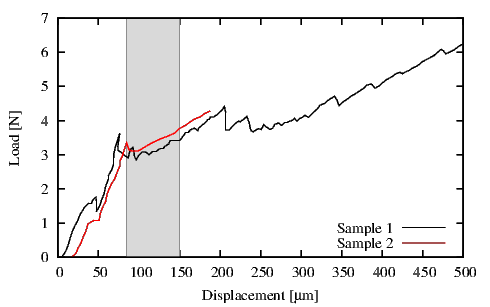Figure 4.25: Experimental results for Sample 1 and Sample 2. The gray area indicates the steady state region where delamination appears.

The Fraunhofer Institute for Electronic Nano System (Fraunhofer-ENAS) used a 4PB specimen  to determine the experimental Gc. In Figure 4.24 the 4PB setup is illustrated. The technique consists of a bimaterial flexural beam with a notch in the top layer. For the bimaterial beam two Si wafers were used to sandwich the film of interest. Under the Si top layer, indicated with h1 in Figure 4.24, a SiO2 layer with a thickness of 500 nm was placed. Subsequently, at the SiO2 film a TiN layer with a thickness of 11 nm and a W layer with a thickness of 200 nm were placed. On the top of the h2 Si layer an adhesive was laid in order to link to the top h1 layer. At the interfaces of three samples an additional Ti layer with a thickness of 25 nm was deposited between the SiO2 and TiN. The deposition process of the TiN layer, the thickness of the layers, and the samples including are presented in Table 4.4. At the end of the experimental measurements the percentage of delamination in the sample was measured (cf. % Del. in Table 4.4). All the samples have a length w of 44 mm and a depth b of 3.5 mm.

 Sample h1 [μm] h2 [μm] % Del. Comments 1 726.3 684.6 28 CVD-TiN with Ti 2 725.7 684.4 7 CVD-TiN without Ti 3 725.5 688.3 78 CVD-TiN with Ti 4 726 698.7 89 CVD-TiN without Ti 5 724.7 683.3 84 PVD-TiN with Ti

Table 4.4: Geometry of the samples used in the simulation study. The thickness h2 includes the thickness of the adhesive. The comments indicate the type of deposition process and the presence or absence of the Ti layer.

In the 4PB method a constant load F and two fixed points are applied at the sample as shown in the Figure 4.24. By recording the load as a function of displacement a plateau was obtained when the crack interface reached the steady state. In this regime G is independent of the crack length and indicates the Gc value .

In the 4PB technique the Euler-Bernoulli beam theory in plane strain condition is applied  and the critical energy release rate Gc is calculated using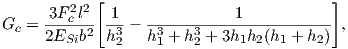(4.65)

where Fc is the force measured at the steady state of the plots in Figure 4.25 and Figure  4.26, ESi is the Young modulus of Si, and the remaining parameters are indicated in Figure 4.24.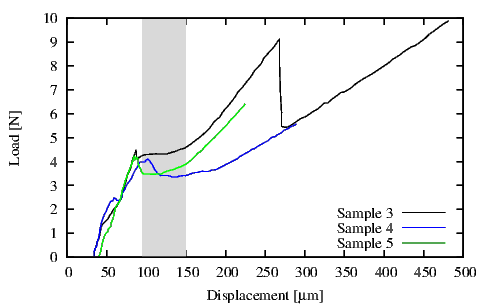Figure 4.26: Experimental results for Sample 3, Sample 4, and Sample 5. The gray area indicates the steady state region where delamination appears.

#### 4.5.2 Simulation

The 4PB technique was simulated using the finite element method. The geometry used for the experiment has been reproduced in two-dimensional simulations. Due to the symmetry conditions in the 4PB test, it is sufficient to consider only half of the structure as depicted in Figure 4.27. All the materials are assumed to be linear elastic. On the left side of the Figure 4.27 a symmetry condition was applied and a fixed point and a load point were applied as in the experiment. Near the crack tip, a very fine triangular mesh was used. The initial conditions for the simulations include an interface crack with a length of 1 mm, which has been increased by 1 mm steps during the simulation until the desired value is reached.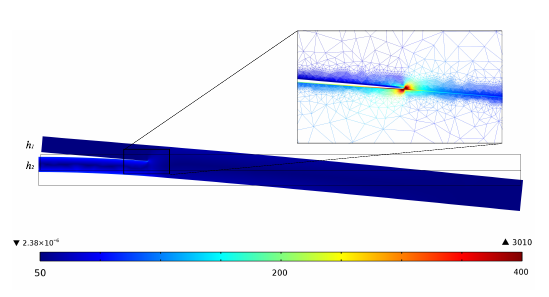Figure 4.27: FEM simulation of the 4PB test. The legend indicates the Von Mises Stress (106 N/m2). Due to the boundary condition the stress development in the bottom layer can be observed. In the inset a zoomed-in section of the crack, with the mesh used is shown.

In Figure 4.27 the results of a simulation with a=5 mm are shown. Due to the boundary condition the Von Mises Stress is mainly generated at the h2 layer.Figure 4.28: Example of the obtained FEM results compared with the stresses calculated using (4.40). Here σxx as a function of r is shown.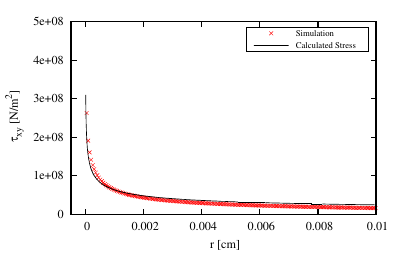Figure 4.29: Example of the obtained FEM results compared with the stresses calculated using (4.40). Here τxy as a function of r is shown.

The stress distribution at the interface crack opening is described by (4.37). For every crack length the σyy and τxy values were extracted as a function of r. Figure 4.28 and Figure 4.29 show an example of the obtained results, where the cross dots are the values of the stresses obtained from the FEM simulations. The x-axis indicates the interface distance at the right side of the crack-tip (r) and the y-axis the stress value. Here, the singularity described in Section 4.1.2 can be observed for both stresses.

#### 4.5.3 Simulation Results

The simulation results are obtained by using the approach described in Section 4.3.2 where the values of σyy and τxy were extracted from the FEM simulations. The G values are calculated by applying the approach for every simulated crack length in the range from 1 mm to 10 mm.

In Figure 4.28 and Figure 4.29 the results of the regression analysis are compared with the FEM simulation results. By using the approach described in Section 4.3.2 the values of K1 and K2 were obtained; by subsequently inserting them in (4.40) the stresses at the interface can be reproduced. The reproduced stresses qualitatively and quantitatively match the FEM simulation results very closely.

Figure 4.30 displays the G values calculated at every crack length a for the Sample 1 and Sample 2 (Table 4.4). As the crack approaches the fixed point of the 4PB test (4 mm from the center of the samples, m in Figure 4.24) the G value decreases .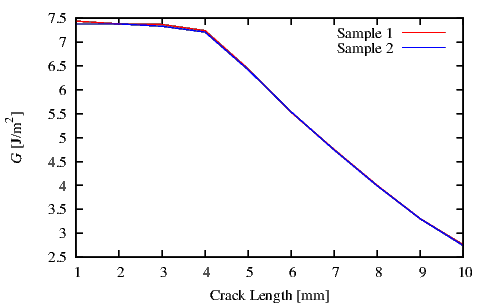Figure 4.30: G obtained from FEM simulations. Crack length in the range from 1 mm to 10 mm was simulated.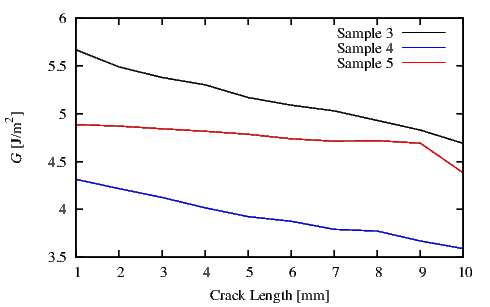Figure 4.31: G obtained from FEM simulations. The crack length in the range from 1 mm to 10 mm was simulated.

The simulated value G at every crack length a for Sample 3, Sample 4, and Sample 5 (Table 4.4) is shown in Figure 4.31. Here the decrease in G is less pronounced than in Sample 1 and Sample 2. The value m=10 mm (same as in the experiment) was used and the simulations were executed until the crack length of a=10 mm was reached. The different line slopes are due to the different thicknesses and interfaces of the analyzed sample.

The experimental Gc was determined by using the average of the loads in a steady state range. In addition, for the FEM simulation results an average value for Gc was determined. In particular for Sample 1 and Sample 2 Gc was calculated in a range of crack lengths from 1 mm to 4 mm and for Sample 3, Sample 4 and Sample 5 in a range of crack lengths from 3 mm to 9 mm.

 Sample Experimental Gc[J∕m2] Simulation Gc[J∕m2] % Error 1 5.95 7.36 19,2 2 5.9 7.33 19,5 3 4.6 5.10 9,8 4 3.5 3.88 9,8 5 3.9 4.75 17,9

Table 4.5: Gc values measured from experimental data and calculated using FEM simulations. The Gc values calculated for Sample 3, Sample 4, and 5 are in good agreement with the experimentally measured Gc values.

In Table 4.5 the Gc values obtained from experimental and simulation data are compared. For Samples 1 and 2 the FEM simulation results differ from the experiments results. This difference is attributed to the behavior observed in Figure 4.25. In these load-displacement plots no clean steady state regions can be observed. The discontinuous sections of the load plots are a result of an unstable crack propagation. It is quite possible that due to some defects or imperfect adhesion between the layers the delamination propagates unevenly. This is also understandable considering the percent of delamination in these two samples (cf. Table 4.4). For Sample 1 and Sample 2 the percentage of delamination is 28 % and 7 %, respectively. For Sample 3, Sample 4, and Sample 5 the percentage of delamination is over 75 % that indicates a stable crack propagation. The FEM simulation results for Sample 3, Sample 4, and Sample 5 appear closer, as given in Table 4.5. The Gc values for Sample 3 and Sample 4 are very close to the experimental values. This is again due to a better adhesion in a longer region and thus a more pronounced steady state load area. In Figure 4.26 a distinguishable constant load created as a result of the delamination is observed. The FEM simulation results the Sample 5 is less accurate but still in an acceptable range (cf. % Err. in Table 4.5).

Simulation results and experimental data imply that the influence of the additional Ti layer (Table 4.4) is negligible.

#### 4.5.4 Discussion

A FEM simulation method to calculate the energy release rate G at the interface between two materials was presented. The 4PB technique can be simulated by considering a simplified structure. From the FEM simulations, the stress at the interface was obtained and used to calculate the energy release rate G. The results of FEM simulations strongly depend on the quality of the experimental data. For the calculation of Gc it is necessary to start with a load-displacement plot, where a steady state load is clearly observable and distinguishable. This study demonstrated the efficiency of the FEM simulation and how it can be used to calculate G for different boundary conditions and geometries of the TSV structure, where the delamination is a potential reliability issue.

The results obtained are in good agreement with experimental measurements. Therefore, the developed model provides a convenient tool for the study of delamination issues in TSVs.

### 4.6 Comparison between J-Integral and Regression Analysis

In the previous sections two different methods for the calculation of the energy release rate G were presented, namely J-Integral and regression analysis. The results in Section 4.5 show good agreement between experimental data and simulations. Therefore, the developed method correctly represent the physical delamination behavior.

A comparison between the two methods is given in this section. Using an identical structure G was calculated with the two methods. The results of the two methods are compared to verify their quality with respect to the G value.

#### 4.6.1 Structure

Figure 4.32 depicts the structure under simulation. At the left side of the structure a symmetric condition was set. Layer 1 indicates Si and layer 2 indicates SiO2 films. Si has a thickness of 70 μm and SiO2 of 72.6 μm. The length w was set to 2.22 mm and the applied force F has a value of 0.2 N. The fixed point was positioned at m=1 mm from the left side of the structure.

As used previously in the other simulations also here a stationary simulation was performed. Crack lengths in the range from 0.1 mm to 0.9 mm were simulated.

Figure 4.32: Structure used for a comparison between the two methods.

#### 4.6.2 Results and Discussion

In Figure 4.33 the simulation results are presented. There is a clear agreement between the two methods. The black crosses indicate the results obtained from the J-Integral method and the red crosses are the results obtained from the regression analysis. Both methods give a constant G for every simulated crack length.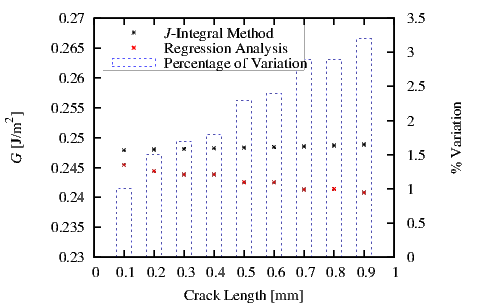Figure 4.33: G values obtained using the J-Integral method and the linear regression method. Crack lengths in the range from 0.1 mm to 0.9 mm were simulated. The percentage of variation between the two methods is reported.

The two methods were verified for the same structure. The results are effectively identical. As shown in Figure 4.33 the variation in percentage between the two methods varies from 1% at small crack lenghts to 3.2% at long crack lengths. The regression analysis becomes less accurate as the simulated crack lengths approach the fixed point m of the structure . The J-Integral method is implemented in a FEM tool. This method permits to calculate the G value directly at the end of the simulation. The method needs to calculate an integral over a path between the two materials. If the material thickness of one layer or both is too thin, the inability of defining a path for the integral can be encountered.

The regression analysis can be applied when thin films are taken in account. It produces the same results, but the evaluation time necessary to calculate G is higher, as the results have to be post processed. After the simulation, the stress at the interface needs to be collected and used in a statistical analysis software, which is used to perform the post-processing fitting.

### 4.7 Summary

In this chapter the delamination theory was explained and the probability of delamination at several material interfaces, essential for the fabrication of an open TSV was investigated.

The energy release rate G, which is an important measure for delamination prediction, was calculated at different interfaces. By comparing G with the critical energy release rate Gc predictions about the probability of delamination can be made. Two different methods used in calculations of G were implemented using FEM. The J-Integral method permits to obtain G at the end of the FEM simulation. The regression analysis method, however requires two post-processing steps. In the first step the obtained stress values at the interface between the two materials need to be extracted. In the second step, through a regression analysis of the collected stress values K1 and K2, necessary to calculate G are determined.

The second method was verified by replicating experiments and the obtained results sufficiently reproduce the measured results. Therefore, the two methods were employed to calculate G for the same structure. The results obtained from the two methods are in good agreement.

While investigating the material interfaces present in open TSVs using the J-Integral method, it was found that the most critical conditions were at the SiO2/W interface. For this interface the Gc was determined experimentally (Section 4.5) and the simulated value (3.5-5.95 J/m2) was higher than that found in literature (0.2-0.5 J/m2 ). This difference is caused by a particular configuration of the film/substrate geometry. The thickness of the layers, the residual stress of the layers, and the employed method to calculate Gc produce different results. Open TSVs have sophisticated structures which do not permit to know the critical condition for the failure. Therefore we are able to qualitatively predict the most unstable condition for delamination by comparing G with the lowest Gc found in literature.

The SiO2/W interface is fundamental for the mechanical stability of open TSVs. G was calculated by investigating the influence of factors such as residual stresses in the films, thicknesses of the layers and, force applied to the system. It was determined, that the most unstable system is one where the residual stress in the W layer is very high, a high external force is applied, or the W film is very thin. Therefore, in the next chapter the origin of the residual stress in solid films is studied.